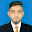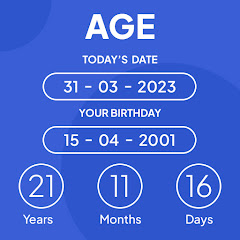# Age calculation - how old am I

4.1
13.8K reviews
500K+Everyone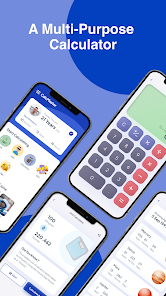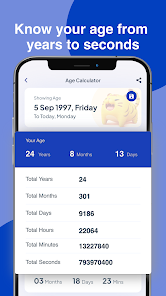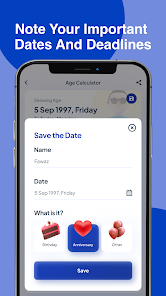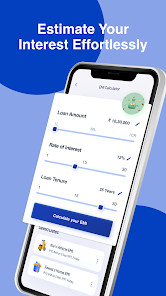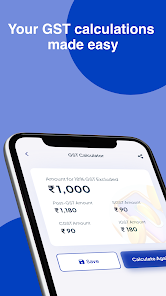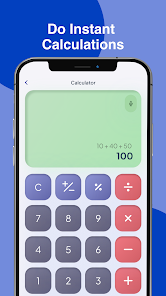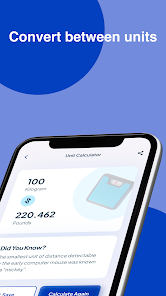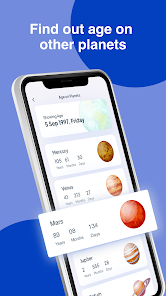Welcome to Pro Calculator- Age Calculator App. Calculate your age from anywhere and anytime calculate age by Date of birth and know you're exact age in years, days & months.

Pro Calculator - The age calculator app by Oogway App is one of the best age calculator apps. If you want to know your exact age in years, months, days, hours, minutes, and seconds, then Download this age calculator app now. Age Calculator is just not just the age calculator app but it helps you to calculate your Pet's age, GST Taxation, Units, Mathematical Calculations, etc.

Ever thought about how old I am? Age Calculator helps you to calculate your or your friend's age using age Calculator Master! You can calculate how old I am to date.

It's not just about an age calculation, you can calculate anything just in a single tap. From EMI Calculator, and GST Calculator to changing inches to meters with unit conversion. All in a single app.

You can also use this app for casio calculator, as Calculator to calculate add separate multiply and divide calculator all basic functions with the full accuracy of a calculator. Calculate all you want what you want

Start the calculator & calculate the age of anything from 1 date to other. Not just that you can use unit conversions to convert one unit of measurement to another, but also calculate your TAX GST amount so that you can know how much GST you would have to pay. You can also calculate the Interest on any bank loan and how much EMI you got to pay for the loan you have taken.

Tutorial

1. Open the Calc master age calculator app
2. You will see a lot of options, you can choose according to your need. There will be options that will help you to calculate pet age calculators, calculate units, EMI on loans, GST,& more. Choose the age calculator option.
3. Now, you know whose age you want to calculate. If you want to calculate your age then enter all the asked accurate details with no mistakes to get an accurate result. If you want to calculate the age of your friend then enter his details correctly. Just like this enter details of whosoever age you want to calculate.
4. Finally! Get your accurate results within seconds.

Features

1. Pet age calculator: This will let you calculate the age of your pet or your friend’s pet, basically any pet animal’s age.
2. EMI Calculator: Age Calculator App helps you to calculate the EMI as well.
3. GST Calculator: Want to calculate GST taxes, this is the app you should calculate on.
4. Unit Calculator: Calculate units, Convert Celsius, Kelvin, Fahrenheit, millimeter, meter, killi meter, etc.
5. Calculator: Use our simple calculator to calculate any mathematical number.

This is how it works! Easy to use and understand. Make it your best buddy, as Age Calculator is made with the best UI, features, and more and it is made by keeping every age group in mind so anyone can use it with ease! Try Age Calculator for yourself to calculate age and you will know how specifically it works!

Calculate what you want where you want, and calculate AGE by Date of birth,

Love the Age Calculator app? Share it with your friends.

Feel free to reach out to us at oogwayapps@gmail.com if you have any questions or pointers related to Calc Master Age Calculator. We’d love to hear from you!
Updated on
Jun 1, 2023

## Data safety

Safety starts with understanding how developers collect and share your data. Data privacy and security practices may vary based on your use, region, and age. The developer provided this information and may update it over time.This app may share these data types with third parties
App info and performance and Device or other IDsThis app may collect these data types
Personal info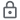Data is encrypted in transit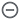Data can’t be deleted

## Ratings and reviews

4.2
13.8K reviews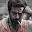Arindam G.
May 13, 2023
It's a great app to calculate Age,date & EMI i am totally impressed by this & I find this very helpful
14 people found this review helpful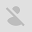Chandamama P
May 6, 2023
It's not good .... Ads are more... because of seeing ads i think installed not for calculating the age...it's too irritating
43 people found this review helpful